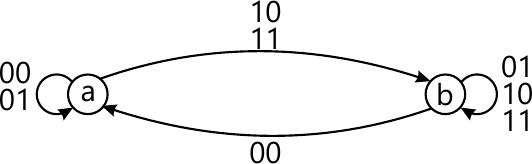MORE IN Software Engineering
VTU Computer Science (Semester 4)
Software Engineering
December 2014
Total marks: --
Total time: --
INSTRUCTIONS
(1) Assume appropriate data and state your reasons
(2) Marks are given to the right of every question
(3) Draw neat diagrams wherever necessary

1(a) What are universal gates? Realize $\left (\overline{(A + B)\cdot \ (\overline{A } +\overline{B})} \right )$ using only universal gates.
5 M
1(b) Discuss the positive and negative logic and list the equivalences in positive and negative logic,
5 M
1(c) An asymmetrical signal waveform is high for 2 m sec and low for 3 m sec.find
i) Frequency
ii) Period
iii) Duty cycle high.
5 M
1(d) Explain the structure of VHDL / Verilog program.
5 M

2(a) The system has four inputs,the output will be high only when the majority of the inputs are high.Find the following :
i) Give the truth table and simplify by using K -map.
ii) Boolean expression in[sum m and prod M form.]
iii) Implement the simplified equation using NAND NAND gates and NOR - NOR gates.
10 M
2(b) Find essential prime implicants for the Boolean expression by using Quine McClusky method.
$f(A,B,C,D)-\sum M (1,3,6,7,9,10,12,13,14,15).$
10 M

3(a) IMPLEMENT THE Boolean function expressed by SOP :
[f(A,B,C,D)-sum - m (1,2,5,6,9,12)] using 3 - to - 1 MUX.
6 M
3(b) Implement a full adder using a 3 - to - 8 decoder.
6 M
3(c) Design 7 - segments decoder using PLA.
8 M

4(a) Give state transition diagram of SR,D,JK and T Filp - flop.
6 M
4(b) With a neat logic diagram and truth table,explain the working of JK Master - slave flip flop along with its implementation using NAND gates.
7 M
4(c) Show how a D flip - flop can be converted into JK flip - flop.
7 M

5(a) With a neat logic diagram,explain the working of a 4- bit SISO register.
10 M
5(b) Design two 4-bit serial Adder.
6 M
5(c) Write the verilog code for switched tail counter using "assign" and "always" statement.
4 M

6(a) Design synchronous mod -5 UP counter using JK Filp-Flop.
10 M
6(b) Explain a 3-bit binary Ripple down counter,give the block diagram,truth table and output waveforms.
10 M

7(a) with a neat block diagram,explain Mealy and Moore model.
10 M
7(b) Design an asynchronous sequential logic circuit for state transition diagram shown in fig.Q7 (b)10 M

8(a) Explain the R/2R Ladder technique of D/A conversion.
10 M
8(b) Explain with neat diagram,single slope A/D converters.
10 M

More question papers from Software Engineering